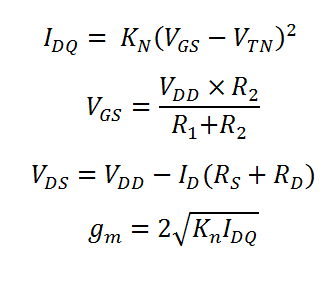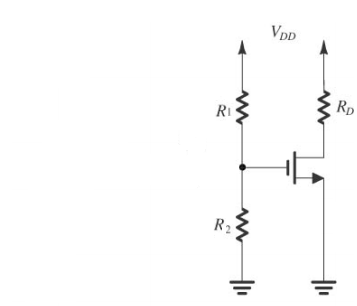# DC Analysis of a Self-Biased CS MOSFET Circuit in saturation mode

This CalcTown calculator calculates the DC parameters like the IDQ and VDS values of a Common Source (CS) self-biased MOSFET circuits, with the MOSFET working in the saturation mode.

*Please note that the body effect has been neglected.

V
uA/V2
K-Ohm
K-Ohm
K-Ohm
V

#### Result

uA
uA/VClick here to view image

where

Kn=1/2(k`W/L)=MOSFET constant

gm=Small signal transconductance